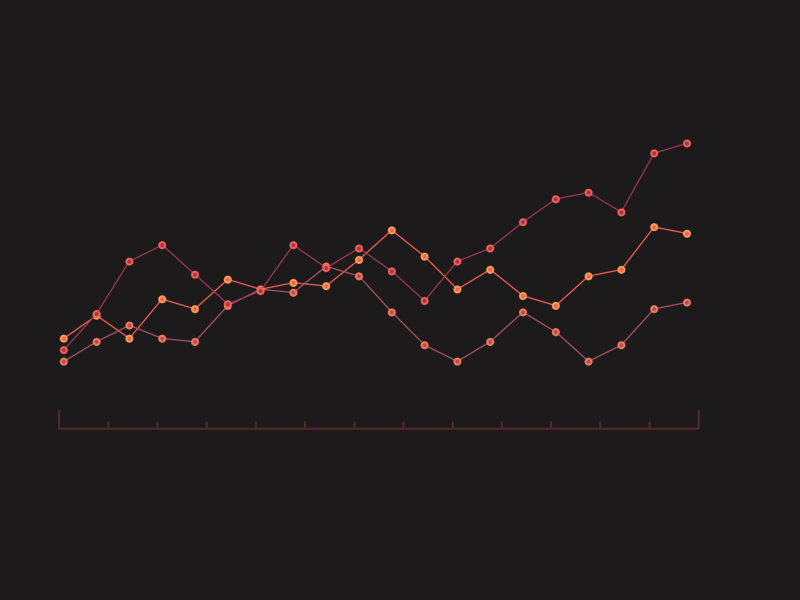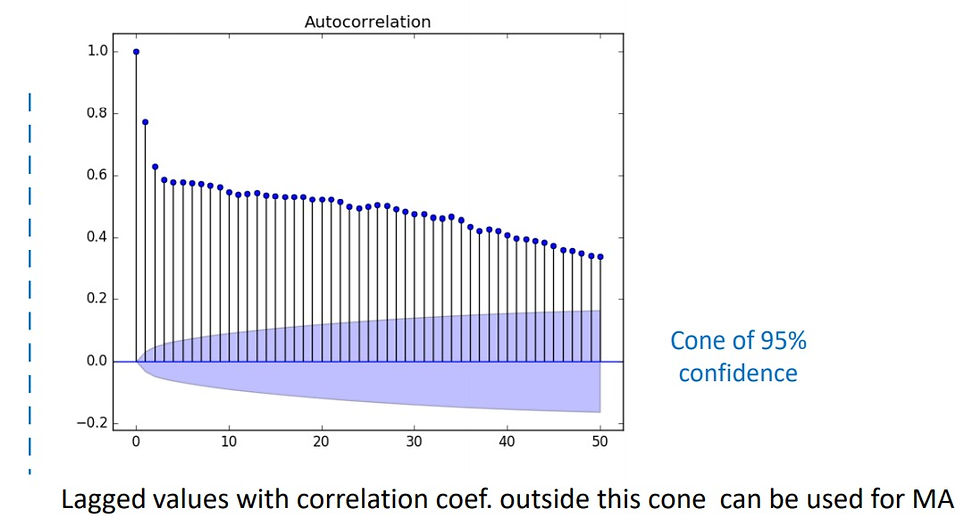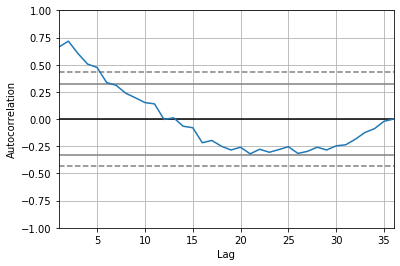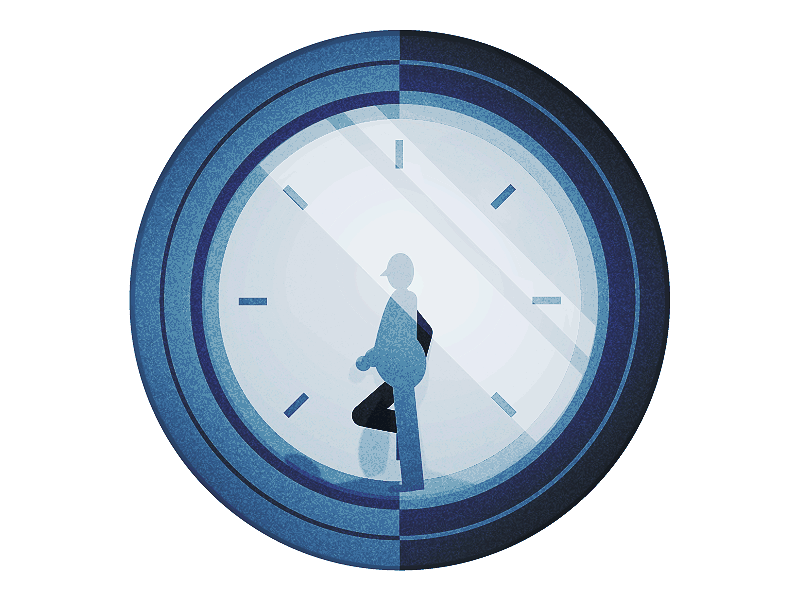top of page
Search
• Dibyajyoti Jena

# Time Series Implementation ModelsBy now, we should have a basic understanding of what a Time Series Dataset is and how to use it. The two main goals of Time Series Analysis are mentioned below.

• Recognizing Data Patterns

• Using the Insights to Make Predictions

Identifying Data-Patterns entails figuring out how the variables are associated, and then using that knowledge to forecast future values.

Models that are most appropriate for the Time Series

In Python and R, there are various Data Science and Machine Learning libraries that include several models for solving Time Series Analysis problems.

The following are some of the most popular Time Series Analysis models:

• ARIMA Models

• SARIMA Models

• Box-Jenkins Multivariate Models

• Holt-Winters Exponential Smoothing

• Unobserved Components Model

ARIMA model• ACF and PACF

In the auto regression model, I tried to find the relationship between the decent value of the variable and the historical values of the variable. But we also had a question: how do we know how many lag values or historical values we should use?

This is where autocorrelation and partial autocorrelation will help us.

Correlation between two variables is basically a relationship or a connection between two numbers. It is measured by Pearson's correlation coefficient, which ranges between minus one to one. If the value of this number is one, this means that the relationship between the two variables is positive. Positive means that if X increases, Y also increases. And if X decreases, Y also decreases.

On the other extreme is if the value is minus one. It means that if X increases Y decreases and if X decreases, Y increases, that both will move in the opposite direction but both are still correlated. There is a relationship between the two, but correlation is negative.

But when the correlation coefficient is zero, this means that there is no relationship between these two variables. So we cannot say that if X increases, what will happen, then Y it may increase or it may decrease.

But when it comes to Time series, we are trying to find the correlation of the variable with its own lag values, which is why, like auto regression, this correlation is called autocorrelation. That is correlation with itself, its own lag values.

To use it, we first find the correlation with all the lag values.ACF (Autocorrelation Function) Plot : The lag values are on the x axis. So this first line is at zero that is, it is for lag zero. The second line is for lag one and so on. On the Y axis, we have correlation coefficient value. So with lag's zero value, that is with itself, the correlation coefficient is plus one, which is obvious with lag one value. It is nearly 0.8.

The colored cone at the bottom is called 95 percent confidence interval cone.

Basically, a point outside this cone means that we are more than 95 percent confident that there is a correlation between these variables.

When we are finding correlation between original series and lag 3 values, we should

remove the effect of lag one and like two values. When we remove these effects of intervening observations, then the correlation coefficient is called partial autocorrelation coefficient.PACF (Partial Autocorrelation Function) Plot

• ARIMA (Auto Regression Integrated Moving Average) Model

Steps :

1. Differencing method is used to remove trends

2. Auto regression is used on the new series to find the initial set of forecasts

3. Moving average model is applied on residuals to update the forecast

4. In the end, differencing is done, that is adding back the lag values to the forecast.

Parameters :

1. AR - p - Order of auto regression(how many lag variables to choose)

2. I - d - Order of Integration (Number of differencing needed)

3. MA - q - Order of moving average (window size for moving average)

• ARIMA model in Python :

Month Sales 1-01 266.0

1-02 145.9

1-03 183.1

1-04 119.3

1-05 180.3

`df['Sales'].plot()`Trend is Polynomial. There is no seasonality

D =2

• Autocorrelation Plot

```from pandas.plotting import autocorrelation_plot
autocorrelation_plot(df['Sales'])```• Partial Autocorrelation Graph

```from statsmodels.graphics.tsaplots import plot_pacf
plot_pacf(df['Sales'], lags=15)```p = 2

```from statsmodels.tsa.arima_model import ARIMA
model = ARIMA(df['Sales'], order=(5,2,2))
model_fit = model.fit()
model_fit.summary()```

ARIMA Model Results

Dep. Variable: D2.Sales No. Observations: 34

Model: ARIMA(5, 2, 2) Log Likelihood -189.034

Method: css-mle S.D. of innovations 54.343

Date: Tue, 03 Mar 2020 AIC 396.067

Time: 17:25:28 BIC 409.805

Sample: 2 HQIC 400.752 coef std_err z P>|z| [0.025 0.975]

const 0.9167 0.256 3.574 0.001 0.414 1.419

ar.L1.D2.Sales -2.1873 0.184 -11.887 0.000 -2.548 -1.827

ar.L2.D2.Sales -2.1231 0.405 -5.246 0.000 -2.916 -1.330

ar.L3.D2.Sales -1.6013 0.478 -3.353 0.002 -2.537 -0.665

ar.L4.D2.Sales -1.0317 0.409 -2.520 0.018 -1.834 -0.229

ar.L5.D2.Sales -0.3193 0.193 -1.653 0.110 -0.698 0.059

ma.L1.D2.Sales -0.0002 0.120 -0.002 0.998 -0.235 0.234

ma.L2.D2.Sales -0.9998 0.120 -8.363 0.000 -1.234 -0.765

Roots

Real Imaginary Modulus Frequency

AR.1 0.1409 -1.3030j 1.3106 -0.2329

AR.2 0.1409 +1.3030j 1.3106 0.2329

AR.3 -1.1313 -0.4225j 1.2076 -0.4431

AR.4 -1.1313 +0.4225j 1.2076 0.4431

AR.5 -1.2502 -0.0000j 1.2502 -0.5000

MA.1 1.0000 +0.0000j 1.0000 0.0000

MA.2 -1.0002 +0.0000j 1.0002 0.5000

```residuals = model_fit.resid
residuals.plot()

residuals.describe()```count 34.000000 mean 11.293855 std 65.989793 min -119.295696 25% -31.207155 50% 12.481503 75% 55.127849 max 156.383323 dtype: float64

• Some Variations of ARIMA model

```ARIMA - model = ARIMA(df['Sales'], order=(q,d,p))
Autoregression - model = ARIMA(df['Sales'], order=(p,d,0))
Moving Average Model - model = ARIMA(df['Sales'], order=(0,d,q))```
`output = model_fit.forecast()`
`output`

(array([636.15148334]), array([54.34286347]), array([[529.64142812, 742.66153855]]))

Forecasted value, standard deviation, 95% confidence interval

`model_fit.forecast(5)`

array([636.15148334, 709.19180983, 664.3330911 , 771.11928552, 761.60381712])

• Walk Forward Validation of ARIMA Model

Since training of statistical models isn't time consuming, walk-forward validation is the most preferred solution to urge most accurate results.

```train_size = int(df.shape*0.7)
train, test = df.Sales[0:train_size], df.Sales[train_size:]
test.shape```

(11,)

```data = train
predict =[]
for t in test:
model = ARIMA(data, order=(5,1,0))
model_fit = model.fit()
y = model_fit.forecast()
print(y)
predict.append(y)
data = np.append(data, t)
data = pd.Series(data)```

387.3764645395876

348.1541436834551

386.30880112815987

356.0820881964668

446.3794710820297

394.73728843470417

434.9154133760461

507.9234715144021

435.48276116299513

652.7439008036883

546.3434721834466

```from sklearn.metrics import mean_squared_error
mse = mean_squared_error(test.values, predict)
mse```

8119.124448295092

SARIMA modelARIMA models cannot handle seasonality in data (ARIMA for only trend in data, SARIMA for both trend and seasonality).

Parameters :

SARIMA(p,d,q)(P,D,Q)m

• p : Trend autoregression order

• d : Trend difference order

• q : Trend moving average order

• P : Seasonal autoregressive order

• D : Seasonal difference order

• Q : Seasonal moving average order

• m : the number of time steps for a single seasonal period

Another extension of SARIMA Model is SARIMAX (Seasonal Auto Regression Integrated Moving Average Exogenous) model. Here we need to input other variables too, known as exogenous variables, other than the variables to be predicted.

For example, to predict stock prices, apart from using historical closing prices, we can also input variables like volume traded, opening price, daily high, etc. We just need to focus that datetime should be kept as an index, and not a separate column.

Implementation in Python

`from statsmodels.tsa.statespace.sarimax import SARIMAX`
`df.head()`

Month MilesMM

0 1963-01-01 6827

1 1963-02-01 6178

2 1963-03-01 7084

3 1963-04-01 8162

4 1963-05-01 8462

```df.index = df['Month']
result_a = seasonal_decompose(df['MilesMM'], model='multiplicative')
result_a.plot()``````model = SARIMAX(df['MilesMM'], order=(5,1,3), seasonal_order=(1,1,1,12))
model_fit = model.fit()
residuals = model_fit.resid
residuals.plot()

``````output = model_fit.forecast()
output```

1971-01-01 11475.842358

Freq: MS, dtype: float64

`model_fit.forecast(12)`

1971-01-01 11475.842358

1971-02-01 11153.512800

1971-03-01 13669.497445

1971-04-01 12647.357108

1971-05-01 14338.979952

1971-06-01 15786.326933

1971-07-01 14979.147877

1971-08-01 15362.201531

1971-09-01 16962.826726

1971-10-01 13682.072572

1971-11-01 12426.861771

1971-12-01 13730.089150

Freq: MS, dtype: float64

```yhat = model_fit.predict()

1963-01-01 0.000000

1963-02-01 5871.999261

1963-03-01 5422.112669

1963-04-01 7122.615626

1963-05-01 7067.315947

Freq: MS, dtype: float64

```pyplot.plot(df['MilesMM'])
pyplot.plot(yhat, color='red')```Final thoughts

One of the most popular Data Analysis issues is Time Series Analysis. There are a number of models and methods that can be used to effectively solve Time Series Analysis problems. Time series forecasting is used in a variety of real-world applications, including:

• Forecasting the Economy

• Forecasting Sales and Marketing

• Estimated Yields

• Predictions from Seismology

• Military Planning